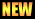# BSc

Notes of Mechanics
Notes of Mechanics written by different authors for BSc or BS.

Notes of Vector Analysis
Handwritten notes of vector analysis for BSc.

Vector Analysis by Hameed Ullah: Notes
All the chapters of vector analysis for BSc made by Hameed Ullah.

Notes of Metric Spaces
Notes of metric spaces for BSc. These notes are related to Section IV of B Course of Mathematics, Paper B.

Notes of Metric Spaces by Umer Asghar
Notes of metric spaces for BSc written by Umer Asghar.

Notes of number theory for BSc. These notes are related to Section II of A Course of Mathematics, Paper B for University of the Punjab.

Notes of Number Theory by Umer Asghar
We are very thankful to Mr. Umer Asghar for sending these notes.

Number Theory by Prof. M. TanveerHandwritten notes of number theory by Prof. M. Tanveer. It include few definitions and basic result necessary for BSc.

Notes by Umer Asghar
Collection of all the notes by Umer Asghar. It include different exercises and chapters for Calculus, Mathematical Method and Number Theory.

Notes of Fourier Series
Notes of fourier series send by Muhammad Ashfaq.

Syllabus and paper pattern
Syllabus and paper pattern for different universities

Formula Pages